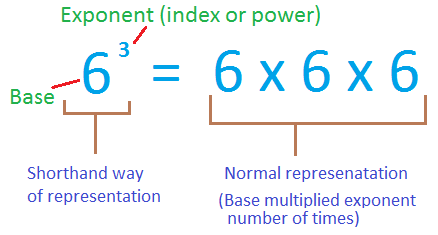1. /
2. CBSE
3. /
4. Class 07
5. /
6. Exponents and Powers worksheet...

# Exponents and Powers worksheet for class 7### myCBSEguide App

Download the app to get CBSE Sample Papers 2023-24, NCERT Solutions (Revised), Most Important Questions, Previous Year Question Bank, Mock Tests, and Detailed Notes.

## Exponents and Powers worksheet for class 7 Important Topics

• Why exponents and powers
• Exponents: The shorthand for repeated multiplications
• Laws of exponents
• Decimal number system and expressing large numbers in the standard form## Some important Facts about Exponents and Powers worksheet for class 7

1.  Very large numbers are difficult to read, understand, compare and operate upon. To make all these easier, we use exponents, converting many of the large numbers in a shorter form.
2. The following are exponential forms of some numbers? 10,000 = 104 (read as 10 raised to 4) 243 = 3 5 , 128 = 27 .
Here, 10, 3 and 2 are the bases, whereas 4, 5 and 7 are their respective exponents. We also say, 10,000 is the 4th power of 10, 243 is the 5th power of 3, etc.

## NCERT class 7Mathematics Solved Worksheets

• Chapter 1 – Integers
• Chapter 2 – Fractions and Decimals
• Chapter 3 – Data Handling
• Chapter 4 – Simple Equations
• Chapter 5 – Lines and Angles
• Chapter 6 – Practical Geometry
• Chapter 7 – The Triangle and its Properties
• Chapter 8 – Congruence of triangles
• Chapter 9 – Comparing Quantities
• Chapter 10 – Rational numbers
• Chapter 11 – Perimeter and Area
• Chapter 12 – Algebraic Expressions
• Chapter 13 – Exponents and Powers
• Chapter 14 – Symmetry
• Chapter 15 – Visualizing Solid Shapes

## EXPRESSING LARGE NUMBERS IN THE STANDARD FORM

Let us now go back to the beginning of the chapter. We said that large numbers can be conveniently expressed using exponents. We have not as yet shown this. We shall do so now.

1. Sun is located 300,000,000,000,000,000,000 m from the centre of our Milky Way Galaxy.
2. Number of stars in our Galaxy is 100,000,000,000.
3. Mass of the Earth is 5,976,000,000,000,000,000,000,000 kg. These numbers are not convenient to write and read. To make it convenient we use powers.

To download Printable worksheets for class 7 Mathematics and Science; do check myCBSEguide app or website. myCBSEguide provides sample papers with solution, test papers for chapter-wise practice, NCERT solutions, NCERT Exemplar solutions, quick revision notes for ready reference, CBSE guess papers and CBSE important question papers. Sample Paper all are made available through the best app for CBSE students and myCBSEguide website.### Test Generator

Create question paper PDF and online tests with your own name & logo in minutes.### myCBSEguide

Question Bank, Mock Tests, Exam Papers, NCERT Solutions, Sample Papers, Notes